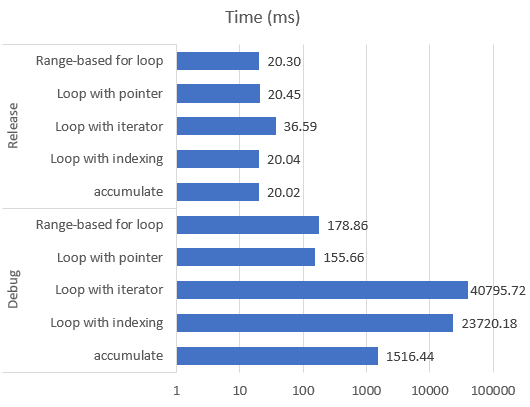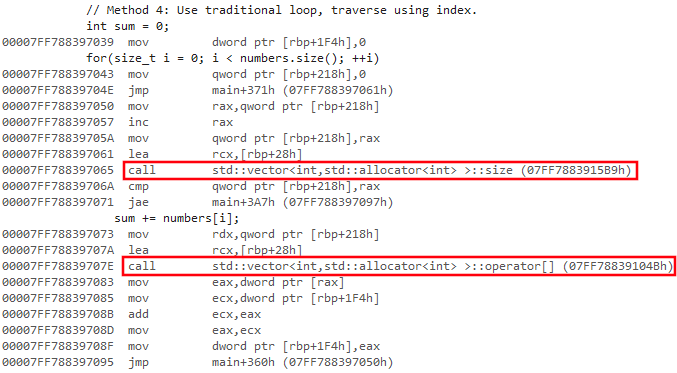# Efficient way of using std::vector

Sat
22
Sep 2018

Some people say that C++ STL is slow. I would rather say it's the way we use it. Sure, there are many potential sources of slowness when using STL. For example, `std::list` or `std::map` tends to allocate many small objects and dynamic allocation is a time-consuming operation. Making many copies of your objects like `std::string` is also costly - that's why I created str_view project. But `std::vector` is just a wrapper over a dynamically allocated array that also remembers its size and can reallocate when you add new elements. Without STL, you would need to implement the same functionality yourself.

When it comes to traversing elements of the vector (e.g. to sum the numerical values contained in it), there are many ways to do it. STL is notoriously known for working very slow in Debug project configuration, but as it turns out, this heavily depends on what method do you choose for using it.

Here is a small experiment that I've just made. In this code, I create a vector of 100,000,000 integers, then sum its elements using 5 different methods, calculating how much time does it take for each of them. Results (averaged over 5 iterations for each method) are as follows. Notice logarithmic scale on horizontal axis.Here is the full source code of my testing program:

```#include <cstdio>
#include <cstdint>
#include <vector>
#include <chrono>
#include <numeric>

typedef std::chrono::high_resolution_clock::time_point time_point;
typedef std::chrono::high_resolution_clock::duration duration;
inline time_point now() { return std::chrono::high_resolution_clock::now(); }
inline double durationToMilliseconds(duration d) { return std::chrono::duration<double, std::milli>(d).count(); }

int main()
{
printf("Iteration,Method,Sum,Time (ms)\n");

for(uint32_t iter = 0; iter < 5; ++iter)
{
std::vector<int> numbers(100000000ull);
numbers = 1; numbers = 2; numbers.back() = 3;

{
time_point timeBeg = now();

// Method 1: Use STL algorithm std::accumulate.
int sum = std::accumulate(numbers.begin(), numbers.end(), 0);

printf("%u,accumulate,%i,%g\n", iter, sum, durationToMilliseconds(now() - timeBeg));
}

{
time_point timeBeg = now();

// Method 2: Use the new C++11 range-based for loop.
int sum = 0;
for(auto value : numbers)
sum += value;

printf("%u,Range-based for loop,%i,%g\n", iter, sum, durationToMilliseconds(now() - timeBeg));
}

{
time_point timeBeg = now();

// Method 3: Use traditional loop, traverse vector using its iterator.
int sum = 0;
for(auto it = numbers.begin(); it != numbers.end(); ++it)
sum += *it;

printf("%u,Loop with iterator,%i,%g\n", iter, sum, durationToMilliseconds(now() - timeBeg));
}

{
time_point timeBeg = now();

// Method 4: Use traditional loop, traverse using index.
int sum = 0;
for(size_t i = 0; i < numbers.size(); ++i)
sum += numbers[i];

printf("%u,Loop with indexing,%i,%g\n", iter, sum, durationToMilliseconds(now() - timeBeg));
}

{
time_point timeBeg = now();

// Method 5: Get pointer to raw array and its size, then use a loop to traverse it.
int sum = 0;
int* dataPtr = numbers.data();
size_t count = numbers.size();
for(size_t i = 0; i < count; ++i)
sum += dataPtr[i];

printf("%u,Loop with pointer,%i,%g\n", iter, sum, durationToMilliseconds(now() - timeBeg));
}
}
}```

As you can see, some methods are slower than the others in Debug configurations by more than 3 orders of magnitude! The difference is so big that if you write your program or game like this, it may not be possible to use its Debug version with any reasonably-sized input data. But if you look at disassembly, it should be no surprise. For example, method 4 calls vector methods `size()` and `operator[]` in every iteration of the loop. We know that in Debug configuration functions are not inilined and optimized, so these are real function calls:On the other hand, method 5 that operates on raw pointer to the vector's underlying data is not that much slower in Debug configuration comparing to Release. Disassembly from Debug version:So my conclusion is: Using std::vector to handle memory management and reallocation and using raw pointer to access its data is the best way to go.

My testing environment was:

CPU: Intel Core i7-6700K 4.00 GHz
RAM: DDR4, Dual-Channel, current memory clock 1066 MHz
OS: Windows 10 Version 1803 (OS Build 17134.285)
Compiler: Microsoft Visual Studio Community 2017 Version 15.4.8
Configuration options: x64 Debug/Release
Windows SDK Version 10.0.16299.0